## A vessel at rest at the origin of an xy coordinate system explodes into three pieces. Just after the explosion, one piece, of mass m, moves

Question

A vessel at rest at the origin of an xy coordinate system explodes into three pieces. Just after the explosion, one piece, of mass m, moves with velocity (-47 m/s) and a second piece, also of mass m, moves with velocity (-47 m/s) . The third piece has mass 3m. Just after the explosion, what are the (a) magnitude and (b) direction (as an angle relative to the +x axis) of the velocity of the third piece?

in progress 0
2 months 2021-07-24T04:54:44+00:00 1 Answers 2 views 0

a) 22.06m/s

b)45°

Explanation:

Let ‘m’ be  mass of 1st and 2nd pieces

mass of 3rd piece=3m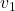=velocity of 1st piece
= -47iˆ  m/s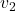=velocity of 2nd piece
= -47jˆ  m/s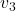=velocity of 3rd piece=?

By considering conservation of linear momentum, we have

m+ m+ 3m=0++ 3=0

3= – (+)= –(+)

Substituting the values ofandin above equation= –(-47iˆ
-47jˆ
) => 15.6iˆ
+ 15.6jˆ

(a)Magnitude of the velocity of  the third piece is given by

|| =√15.6²+15.6² => 22.06m/s

(b) its direction (as an angle relative to the +x axis)  ‘θ’

θ=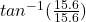=>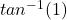=>45°